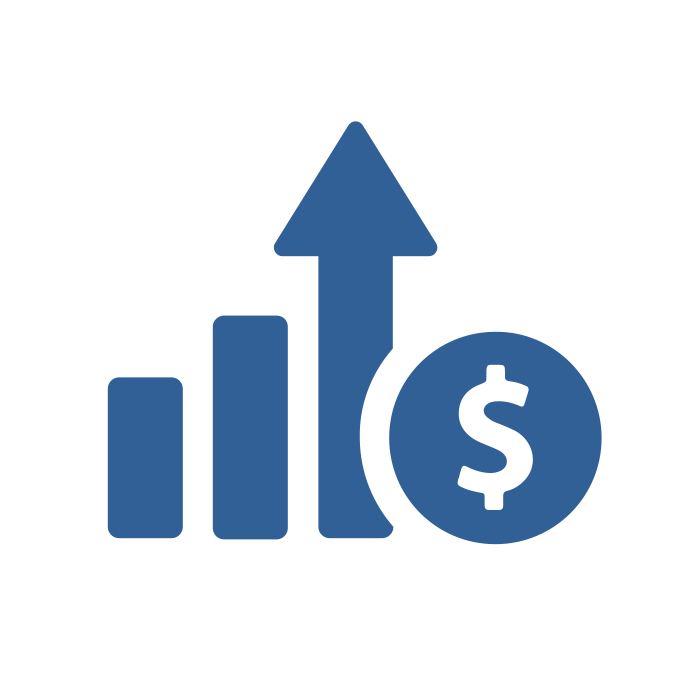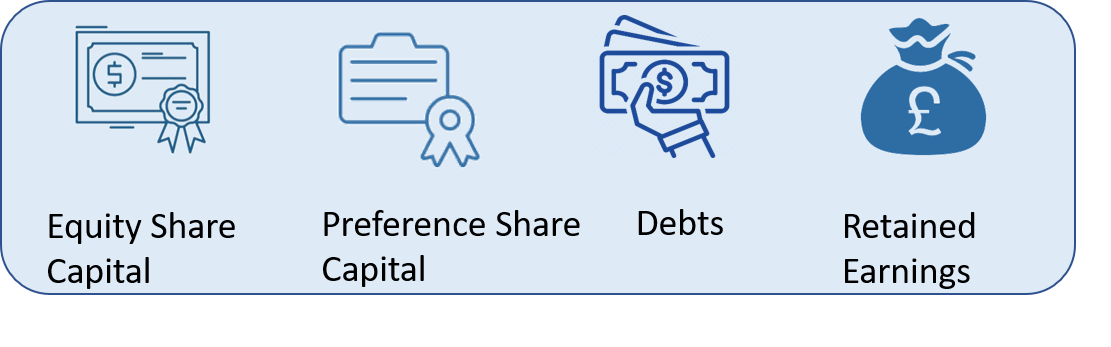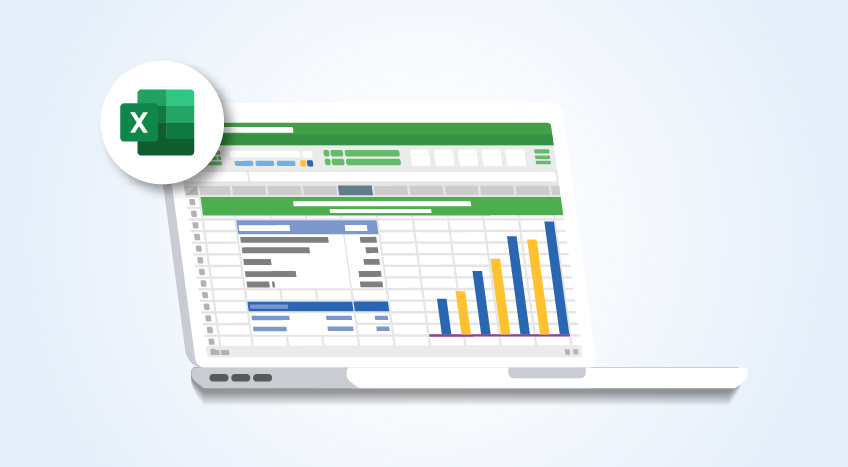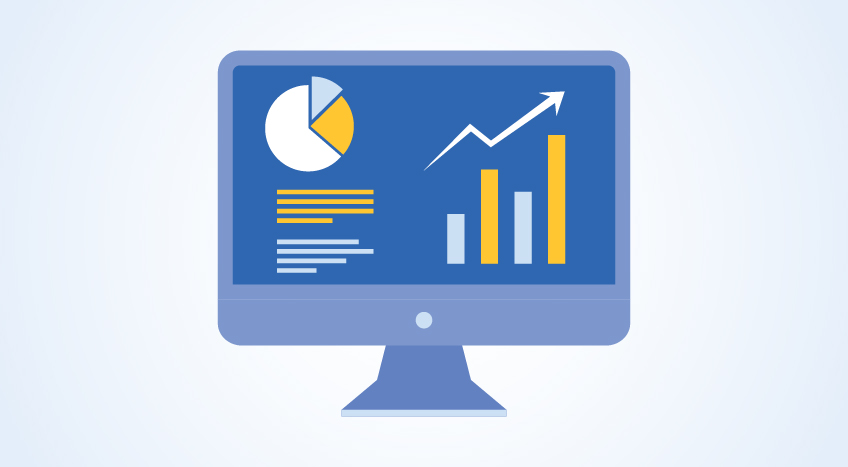# Cost Of Capital – Definition, Formula, Calculation and Example

## Definition of Cost of Capital

Cost of Capital is the rate of return the firm expects to earn from its investment in order to increase the value of the firm in the market place. In other words, it is the rate of return that the suppliers of capital require as compensation for their contribution of capital.## Source of Cost of Capital

The source of capital employed by the firm is usually in the following form:## Components of Cost of Capital

There are three factors to the cost of capital explained below:

### Zero Risk Return

It talks about the expected rate of return when a project involves no financial or business risks.

Business risk is determined by the capital budgeting decisions that a firm takes for its investment proposals. So, if a firm selects a project that has more than normal risk, then it is obvious that the providers of capital would require or demand a higher rate of return than the normal rate.

Thus the premium factor plays an important role here as it increases the Cost of Capital. But how much premium? it’s up to the firm’s project selection decision which alienates with the firm’s goal and objectives and how badly they want the project to increase their market value.

### Premium for the Financial Risk

Financial risk is associated with the capital structure pattern of the firm. Here, the premium finds its way to the picture depending on the volume of debts the firm owes. The higher the debt capital, the more is the risk compared to a firm that has relatively low debts.

## Cost of Capital Formula

The three components of cost of capital discussed above can be written in an equation as follows:

K = Cost of Capital

r0 = Return at zero risk level

2. = Premium for finance risk

## How to Calculate of Cost of Capital

In calculating the cost of capital, the following methods can be used:

1. ### Computation of Specific Cost of Capital

Specific Cost refers to the cost which is associated with the source of capital. Eg. Cost of equity. Computing specific cost of capital involves summing up of all forms of capital listed below

• Cost of debt
• Cost of preference shares
• Cost of equity shares
• Cost of retained earnings
1. ### Computation of Composite Cost of Capital

Composite capital is the combined cost of different sources of capital taken together. It is also called a Weighted Average Cost of Capital (WACC). Following are steps involved in the calculation of WACC. The formula to arrive is given below:

Ko = Overall cost of capital

Wd = Weight of debt

Wp = Weight of preference share of capital

Wr = Weight of retained earnings

We = Weight of equity share capital

Kd = Specific cost of debt

Kp = Specific cost of preference share capital

Kr = Specific cost of retained earnings

Ke = Specific cost of equity share capital

Looks bookish? We have got it simplified with the example.

## Example of Cost of Capital calculations using WACC

Aero Ltd had the following cost capital structure employed for financing its projects and would like to calculate the cost of capital.

 Amount ( Rs. ) After-tax Cost % Equity share capital 8,00,000 16% 0.0225 Retained earnings 4,00,000 15% 0.03 Preference share capital 6,00,000 12% 0.025 Debentures 6,00,000 9% 0.053 Total 24,00,000

Calculation of Cost of capital of Aero Ltd

 Source Amount (Rs. )       (1) Weights (Specific Capital/Total cost)   (2) After-tax Cost (Cost%/100)     (3) Weighted Cost       (4) = (2) *(3) Equity share capital 8,00,000 0.34 0.16 0.053 Retained earnings 4,00,000 0.16 0.15 0.024 Preference share capital 6,00,000 0.25 0.12 0.03 Debentures 6,00,000 0.25 0.09 0.023 Total 24,00,000 0.13

Weight Average Cost of Capital here is 13% (0.13*100). This implies that the overall cost of capital employed by Aero Ltd is 13%. In other words, we can say that the company is paying a premium of 13% to the lenders of capital as a return for their risk.

You can use the formula we discussed, and the result will be similar.

= (6,00,000 / 24,00,000) * 0.09 + (6,00,000 / 24,00,000) * 0.12 + ( 4,00,000 / 24,00,000 ) * 0.15 + ( 8,00,000 / 24,00,000) * 0.16 = 13%

Determining Cost of Capital is one of the key factors in deciding the investment. It helps you in evaluating the different investment projects basis the cost, benefits and risks. Another important factor to be considered here is capital budgeting and payback period. Here, the payback period is nothing, but the time taken to recover the investment amount. Read "What Is Capital Budgeting? Process, Calculation and Example to know the process and calculations.

Language

#### Latest Blogs